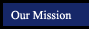Geometry Textbook Tutorials
textbook tutorials - definitions - constructions - postulates and theorems - internet activities and resourcesChapter 1: Reasoning in Geometry Chapter 2: Segment Measures and Coordinate Graphing Chapter 5: Triangles and Congruence Chapter 6: More about Triangles Chapter 7: Triangle Inequalities Chapter 8: Quadrilaterals Chapter 9: Proportions and Similarity Chapter 10: Polygons and Area Chapter 12: Surface Area and Volume Chapter 13: Right Triangles and Trigonometry Chapter 14: Circle Relationships Chapter 15: Formalizing Proof Chapter 16: More Coordinate Graphing and Transformations